##Hyperbola with given asymptotic triangle

Given a triangle ABC, there is exactly one hyperbola tangent to its side AC at its middle M, having the triangle as asymptotic i.e. having B as the center of the hyperbola and the other sides BA, BC as asymptotic lines.

The hyperbola is uniquely determined by one of its points (M) and the location of its focal points D and E. This is done as follows:
- Draw the bisector BD of the angle at B.
- Construct the circle, whose points on the arc CDA view the segment AC under the angle = ang(EBA).
- The intersection-points D, E of this circle with the bisector BD are the focal points of the hyperbola.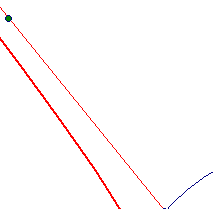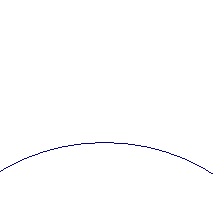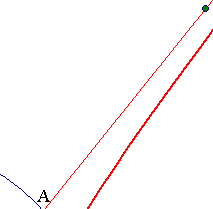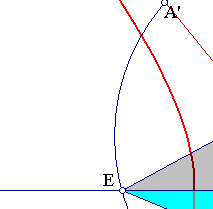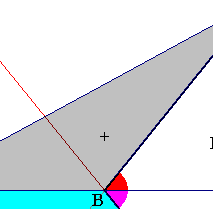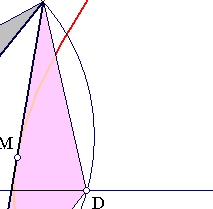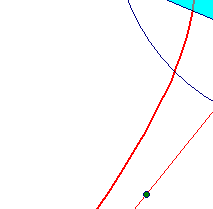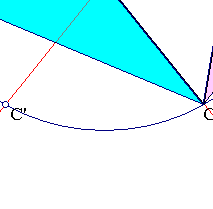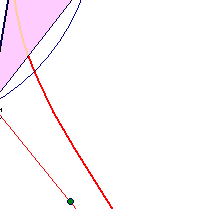From the construction of D, follows that triangles EBA, CDA and CBE are similar. Angles ang(CAD) = ang(EAB), thus EC' = CD and analogously EA' = AD and B is the middle of ED. The construction here is the inverse of the one discussed in the file AsymptoticTriangle.html .

Remark The aformentioned reference and this discussion imply that for a triangle ABC with fixed angle(ABC) and variable AC, but so that the area(ABC) remains constant, side AC envelopes a hyperbola.